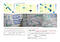# Cancer Diagnosis — Stochastic Gradient Descent Algorithms

In my earlier post regarding Logistic-regression loss minimization we had seen that by changing the form of the loss function we can derive other machine learning models. It’s precisely this that we are going to talk about in this post.

Below is a table that shows a few loss functions and their associated machine learning algorithms.Random forest

# Cancer Diagnosis — Random Forest

We are going to use the same dataset we used in the Personal Cancer Diagnosis artile that was publised earlier

We will not be dealing with the exploratory data analysis but will straight go ahead with the machine learning model implementation of the dataset.

In Random Forests we have

• Decision Trees as base learners
• Row Sampling with replacement
• Column / Feature Sampling
• Aggregation for Label generation

When using Random Forests for

• Classification for the final label generation we will use majority vote
• Regression the mean / median will be calculated for the target value

Base learners are Decision Trees with…

# Personal Cancer Daignosis

## Problem statement :

Classify the given genetic variations/mutations based on evidence from text-based clinical literature.

## Data

Data: Thanks to Memorial Sloan Kettering Cancer Center (MSKCC) for curating this dataset a great help to humanity.

## Business Objective and Constraints

• There is no Latency requirement
• High accuracy is required as we are dealing with human lives
• Errors are very costly
• Interpretability is very important if the AI/ML model needs to be accepted by the Medical Community. The medical community needs to know how the model arrived at the classification rather than being a black-box.
• This being a multi-class classification , the model needs to output probability scores which…

# Logistic Regression — probabilistic interpretation

Let start with the assumptions that we need to make

• The class label Y takes only two outcomes+1, 0 like a coin toss and hence can be thought of as a Benoulli random variable. The first big assumption is that the class label Y has a Bernoulli distribution.
• We have features X= {x1,x2,x3,…xn) where each xi is a continous variable. The next assumption is that the conditional probability of these features are Gaussian distributed. for each xi P(xi|y=yk) is gaussian distributed
• For two features xi and xj where i not equal to j, then xi and xj are conditionally independent…

# Logistic Regression

Logisitc regression ==> simple Algorithm

Naive Bayes ==> No geometric intuition. Learnt it through Probabilistic technique

Logistic Regression can be learnt from three perspectives

• Geometric intuition
• probabilistic approach which involves dense mathematics
• Loss minimization framework

# Assumption

• Classes are linearly or almost linearly seperable plane

# Applications

• Google Translate
• Auto Reply
• Auto Suggestion

Seminal paper — ILya Sutskever

Machine Translation ==> Seq to Seq Models

# Objective

This is a two class label(1 — client with payment difficulties, 0 — all other cases) classification problem.

# Table of Contents

1) Introduction

2) Business problem

3) Mapping to ML problem

4) Introduction to Datasets

5) Existing approaches

6) First Cut Approach

7) Exploratory Data Analysis (EDA)

8) Feature engineering

9) Model Explanation

10) Results

11) Conclusion and Future Work

12) Profile

13) References

# 1)Introduction

This blog is about how to apply technological advances from the field of machine learning to a sector that serves as one of the main pillars of society- the banking sector.

Large industries or common man like to full…## Janardhanan a r

In the making Machine Learner programmer music lover